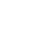Live chata). With an increase in aggregate demand the resulting equilibrium will be at point B, where the AD2 curve intersect with the LRAS curve. The corresponding equilibrium price will be at point P2, this imply an increase in the price level from P1 to P2. The economy will adjust to the initial long run equilibrium at point A by increasing the supply that results in a decline of commodity prices. The supply of the commodity will reduce the price from P2 to P1 returning the economy into its original equilibrium because AD will equal LRAS. The price will fall up to the point where AD equals AS[.

b) An increase in the price of raw material will lead to an increase in the price level of the commodity from the equilibrium price P1 to the new price P2. The new equilibrium point will be at point C. The economy will revert to the original position by increasing production of the commodity whose production cost has increased so as to minimize the cost per unit of production hence lowering the price levels.

## Buy Free Custom «Economics Questions» Essay Paper paper online

* Final order price might be slightly different depending on the current exchange rate of chosen payment system.

Question 12

a) The real GDP will be 1260 billions of dollars and price levels 110.5 dollars.

b) RBA should use an expansionary policy to increase the number of exports. RBA will therefore, have to purchase securities through open market operations.

c)Inflation rate (without policy)= (110.5 -107.0)/ 107.0 * 100

ci)

Stay Connected= 3.27

Inflation rate (with policy) = (109.5- 107.0)/ 107.0 *100

= 2.34

Question 16

a). Real GDP Growth = (2010's Real GDP - 2009's Real GDP) / (2009's Real GDP)
= (1160 – 1120)/ 1120

= 0.035 *100

= 3.5 %

Limited time Offer

0
0
days
:
0
0
hours
:
0
0
minutes
:
0
0
seconds
Get 19% OFF

b). Inflation rate = (1160- 1120)/ 1120 *100

= 3.5

c). Growth rate for 2010 = 1120/ 1160

= 0.96

#### Related Economics essays

0

Preparing Orders

0

Active Writers

0

Support Agents

Limited offerget 15% off your 1st order